‘fourierin’ package

2019-04-04

This is a package in R to numerically calculate Fourier-type integrals of multivariate functions with compact support evaluated at regular grids. Specifically, integrals of the type

$I \left[f(t), \boldsymbol{a}, \boldsymbol{b};r, s \right] = \left[ \frac{|s|}{(2\pi)^{1 - r}}\right]^{n/2} \int_{a_1}^{b_1}\int_{a_2}^{b_2}\cdots\int_{a_n}^{b_n} f(\boldsymbol{t})e^{\imath s \langle \boldsymbol{w}, \boldsymbol{t}\rangle} \text{d}\boldsymbol{t},$

where,

$$\boldsymbol{a} = (a_1, \ldots, a_n)$$, $$\boldsymbol{b} = (b_1, \ldots, b_n)$$, $$\boldsymbol{t} = (t^{(1)}, \ldots, t^{(n)})$$, $$\boldsymbol{w} = (w^{(1)}, \ldots, w^{(n)})$$, $$a_l \leq t^{(l)} \leq b_l$$, $$c_l \leq w^{(l)} \leq c_l$$.

Common values for r are -1, 0 and -1, while common values for s are -2, -1, 1 and 2. For example, if f is a density function, s = 1 and r = 1 could be used to obtain the characteristic function of f. Conversely, if f is the characteristic function of a probability density function, then r = -1 and s = -1 could be used to recover the density.

The implementation of this algorithm is the one described in Inverarity (2002), Fast Computation of multidimensional Integrals.

Some examples (also found in documentation).

Example 0: Speed tests

Below it is an example of univariate continuous Fourier transform.

library(fourierin)
library(dplyr)
##
## Attaching package: 'dplyr'
## The following objects are masked from 'package:stats':
##
##     filter, lag
## The following objects are masked from 'package:base':
##
##     intersect, setdiff, setequal, union
library(purrr)
library(ggplot2)

## Test speed at several resolutions
resolution <- 2^(3:8)

## Function to be tested
myfnc <- function(t) exp(-t^2/2)

## Aux. function
compute_times <- function(resol){
reps <- 100
rbenchmark::benchmark(
yes = fourierin_1d(f = myfnc, -5, 5, -3, 3, -1, -1, resol),
no = fourierin_1d(f = myfnc, -5, 5, -3, 3, -1, -1, resol,
use_fft = FALSE),
replications = reps) %>%
as.data.frame() %>%
select(FFT = test, time = elapsed) %>%
mutate(time = time*1e3/reps, resolution = resol)
}

resolution %>%
map_df(compute_times) %>%
mutate(resolution = as.factor(resolution)) %>%
ggplot(aes(resolution, time, color = FFT)) +
geom_point(size = 2, aes(shape = FFT)) +
geom_line(aes(linetype = FFT, group = FFT)) +
ylab("time (in milliseconds)")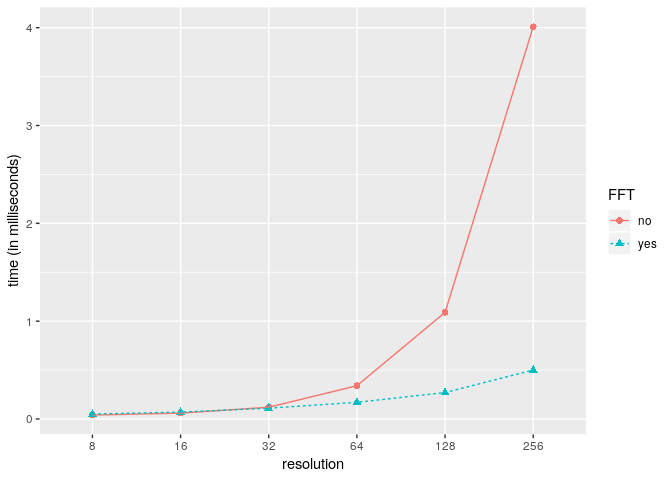Now we present an example using a bivariate function.

library(fourierin)
library(dplyr)
library(purrr)
library(ggplot2)

## Test speed at several resolutions
resolution <- 2^(3:7)

## Bivariate function to be tested
myfnc <- function(x) dnorm(x[, 1])*dnorm(x[, 2])

## Aux. function
compute_times <- function(resol){
reps <- 1
resol <- rep(resol, 2)
rbenchmark::benchmark(
yes = fourierin(myfnc,
lower_int = c(-8, -6), upper_int = c(6, 8),
lower_eval = c(-4, -4), upper_eval = c(4, 4),
resolution = resol),
no = fourierin(myfnc,
lower_int = c(-8, -6), upper_int = c(6, 8),
lower_eval = c(-4, -4), upper_eval = c(4, 4),
resolution = resol, use_fft = FALSE),
replications = reps) %>%
as.data.frame() %>%
select(FFT = test, time = elapsed) %>%
mutate(time = time/reps, resolution = resol)
}

## Values
comparison <-
resolution %>%
map_df(compute_times)

fctr_order <-
unique(comparison$resolution) %>% paste(., ., sep = "x") ## Plot comparison %>% mutate(resolution = paste(resolution, resolution, sep = "x"), resolution = ordered(resolution, levels = fctr_order)) %>% ggplot(aes(resolution, time, color = FFT)) + geom_point(size = 2, aes(shape = FFT)) + geom_line(aes(linetype = FFT, group = FFT)) + ylab("time (in seconds)")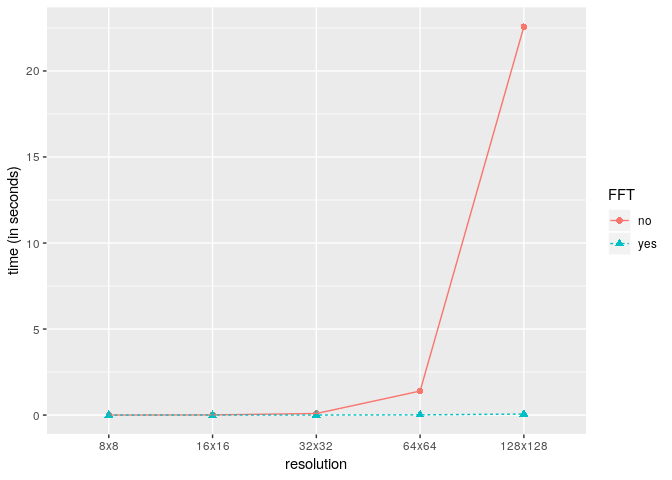Example 1: Recovering standard normal from its characteristic function The density function of a random variable can be recovered using the Fourier inversion theorem. The characteristic function of a standard normal distribution is $\phi(t) = \exp(-t^2/2) \;\; t \in \mathbb{R}$ The due to the smoothness and symmetry of both, the characteristic function and the standard normal density, it can be well approximated even with a low resolution. library(fourierin) library(dplyr) library(ggplot2) ## Characteristic function of std. normal. fnc <- function(t) exp(-t^2/2) ## Compute integral out <- fourierin(f = fnc, lower_int = -5, upper_int = 5, lower_eval = -5, upper_eval = 5, const_adj = -1, freq_adj = -1, resolution = 64) ## Extract values and compute true values of the density df1 <- out %>% as_data_frame() %>% mutate(values = Re(values), density = "approximated") ## Warning: as_data_frame() is deprecated, use as_tibble() (but mind the new semantics). ## This warning is displayed once per session. df2 <- data_frame(w = seq(min(df1$w), max(df1$w), len = 150), values = dnorm(w), density = "true") ## Warning: data_frame() is deprecated, use tibble(). ## This warning is displayed once per session. bind_rows(df1, df2) %>% ggplot(aes(w, values, color = density)) + geom_line(aes(linetype = density)) + xlab("x") + ylab("f(x)")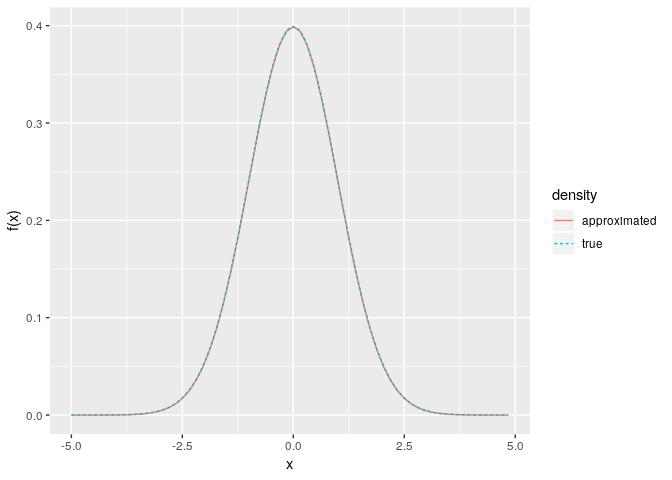We now present a second example: recovering the density of a $$\chi^2$$ distribution using its characteristic function. The corresponding density is not symmetric and its support is not the entire real line, thus a higher resolution might be required to achieve a good approximation. library(fourierin) library(dplyr) library(purrr) library(ggplot2) ## Set functions df <- 5 cf <- function(t) (1 - 2i*t)^(-df/2) dens <- function(x) dchisq(x, df) ## Set resolutions resolutions <- 2^(6:8) ## Compute integral given the resoltion recover_f <- function(resol){ ## Get grid and density values out <- fourierin(f = cf, lower_int = -10, upper_int = 10, lower_eval = -3, upper_eval = 20, const_adj = -1, freq_adj = -1, resolution = resol) ## Return in dataframe format out %>% as_data_frame() %>% transmute( x = w, values = Re(values), resolution = resol) } ## Density approximations vals <- map_df(resolutions, recover_f) ## True values true <- data_frame(x = seq(min(vals$x), max(vals$x), length = 150), values = dens(x)) vals %>% mutate(resolution = ordered(resolution, labels = resolutions)) %>% ggplot(aes(x, values)) + geom_line(aes(color = resolution)) + geom_line(data = true, aes(color = "true values"))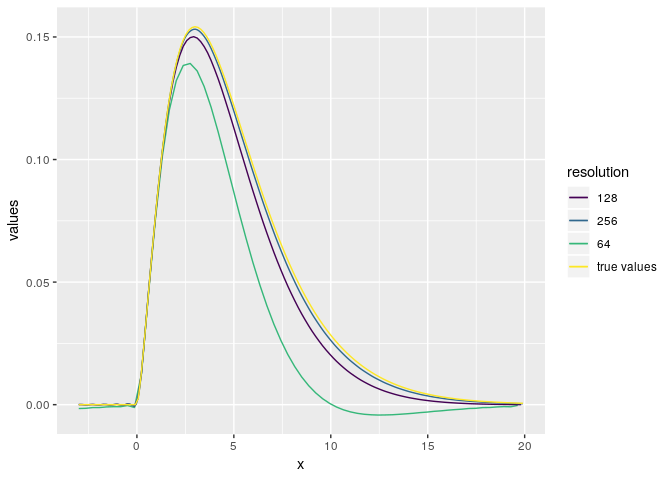Example 2: Computing characteristic function of a gamma density library(fourierin) library(tidyr) library(dplyr) library(purrr) library(ggplot2) ## Compute integral shape <- 5 rate <- 3 myfnc <- function(t) dgamma(t, shape, rate) ## Function to compute characteristic function for a given resolution. approximate_CF <- function (resol) { out <- fourierin(f = myfnc, -0, 8, -5, 5, 1, 1, resol) out %>% as_data_frame() %>% transmute(t = w, real = Re(values), imaginary = Im(values), resolution = as.character(resol)) %>% gather(CF, values, -t, -resolution) } ## Evaluate approxs. to CF for different resulutions resolution <- 2^c(6, 7) CF <- map_df(resolution, approximate_CF) ## Evaluate true values of characteristic function true_cf <- function(t, shape, rate) (1 - 1i*t/rate)^-shape true <- data_frame(t = seq(min(CF$t), max(CF\$t), length = 150),
values = true_cf(t, shape, rate),
real = Re(values),
imaginary = Im(values),
resolution = "true values") %>%
select(-values) %>%
gather(CF, values, -t, -resolution)

## Plot values
CF %>%
ggplot(aes(t, values, color = resolution,
group = resolution)) +
geom_line(aes()) +
geom_line(data = true) +
facet_grid(~CF) +
theme(legend.position = "bottom")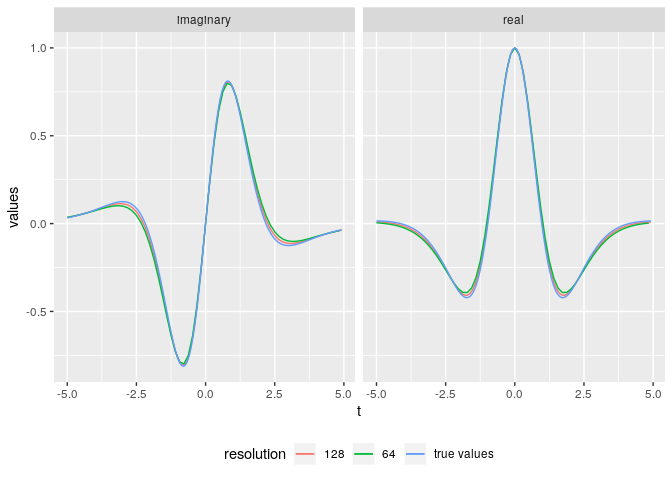Example 3: Recovering std. normal from its characteristic function

Testing example.

library(fourierin)
library(tidyr)
library(dplyr)
library(purrr)
library(lattice)
library(ggplot2)

## Set functions to be tested with their corresponding parameters.
mu <- c(-1, 1)
sig <- matrix(c(3, -1, -1, 2), 2, 2)

## Multivariate normal density, x is n x d
f <- function(x) {
## Auxiliar values
d <- ncol(x)
z <- sweep(x, 2, mu, "-")
## Get numerator and denominator of normal density
num <- exp(-0.5*rowSums(z * (z %*% solve(sig))))
denom <- sqrt((2*pi)^d*det(sig))
return(num/denom)
}

## Characteristic function, s is n x d
phi <- function (s) {
complex(modulus = exp(-0.5*rowSums(s*(s %*% sig))),
argument = s %*% mu)
}

## Evaluate characteristic function for a given resolution.
eval <- fourierin(f,
lower_int = c(-8, -6), upper_int = c(6, 8),
lower_eval = c(-4, -4), upper_eval = c(4, 4),
const_adj = 1, freq_adj = 1, resolution = 2*c(64, 64),
use_fft = T)

## --- Little test
dat <- eval %>%
with(crossing(y = w2, x = w1) %>%
mutate(approximated = c(values))) %>%
mutate(true = phi(matrix(c(x, y), ncol = 2)),
difference = approximated - true) %>%
gather(value, z, -x, -y) %>%
mutate(real = Re(z), imaginary = Im(z)) %>%
select(-z) %>%
gather(part, z, -x, -y, -value)

## Surface plot
wireframe(z ~ x*y | value*part, data = dat,
scales =
list(arrows=FALSE, cex= 0.45,
col = "black", font = 3, tck = 1),
screen = list(z = 90, x = -74),
colorkey = FALSE,
light.source= c(0,10,10),
shade.colors = function(irr, ref,
height, w = 0.4)
grey(w*irr + (1 - w)*(1 - (1 - ref)^0.4)),
aspect = c(1, 0.65))

## Contours of values
dat %>%
filter(value != "difference") %>%
ggplot(aes(x, y, z = z)) +
geom_tile(aes(fill = z)) +
facet_grid(part ~ value) +
scale_fill_distiller(palette = "Reds")

## Contour of differences
dat %>%
filter(value == "difference") %>%
ggplot(aes(x, y, z = z)) +
geom_tile(aes(fill = z)) +
facet_grid(part ~ value) +
scale_fill_distiller(palette = "Spectral")